# 7.5 General strategy for factoring polynomials  (Page 2/2)

 Page 2 / 2

Factor completely: $16{x}^{3}-36x$ .

$4x\left(2x-3\right)\left(2x+3\right)$

Factor completely: $27{y}^{2}-48$ .

$3\left(3y-4\right)\left(3y+4\right)$

Factor completely: $4{a}^{2}-12ab+9{b}^{2}$ .

## Solution

 Is there a GCF? No.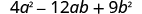Is it a binomial, trinomial, or are there more terms? Trinomial with $a\ne 1$ . But the first term is a   perfect square. Is the last term a perfect square? Yes.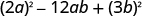Does it fit the pattern, ${a}^{2}-2ab+{b}^{2}?$ Yes.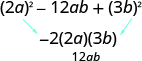Write it as a square.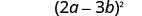Check your answer. Is the expression factored completely? Yes. The binomial is not a difference of squares. Multiply. ${\left(2a-3b\right)}^{2}$ ${\left(2a\right)}^{2}-2\cdot 2a\cdot 3b+{\left(3b\right)}^{2}$ $4{a}^{2}-12ab+9{b}^{2}✓$

Factor completely: $4{x}^{2}+20xy+25{y}^{2}$ .

${\left(2x+5y\right)}^{2}$

Factor completely: $9{m}^{2}+42mn+49{n}^{2}$ .

${\left(3m+7n\right)}^{2}$

Factor completely: $6{y}^{2}-18y-60$ .

## Solution

$\begin{array}{ccccccc}\text{Is there a GCF?}\hfill & & & \text{Yes, 6.}\hfill & & & \hfill 6{y}^{2}-18y-60\hfill \\ \text{Factor out the GCF.}\hfill & & & \text{Trinomial with leading coefficient 1.}\hfill & & & \hfill 6\left({y}^{2}-3y-10\right)\hfill \\ \begin{array}{c}\text{In the parentheses, is it a binomial, trinomial,}\hfill \\ \text{or are there more terms?}\hfill \end{array}\hfill & & & & & & \\ \text{“Undo” FOIL.}\hfill & & & \hfill 6\left(y\phantom{\rule{1em}{0ex}}\right)\left(y\phantom{\rule{1em}{0ex}}\right)\hfill & & & \hfill 6\left(y+2\right)\left(y-5\right)\hfill \\ \\ \\ \text{Check your answer.}\hfill & & & & & & \\ \text{Is the expression factored completely?}\hfill & & & & & & \hfill \text{Yes.}\hfill \\ \text{Neither binomial is a difference of squares.}\hfill & & & & & & \\ \text{Multiply.}\hfill & & & & & & \\ \\ \\ \phantom{\rule{2.5em}{0ex}}6\left(y+2\right)\left(y-5\right)\hfill & & & & & & \\ \phantom{\rule{2.5em}{0ex}}6\left({y}^{2}-5y+2y-10\right)\hfill & & & & & & \\ \phantom{\rule{2.5em}{0ex}}6\left({y}^{2}-3y-10\right)\hfill & & & & & & \\ \phantom{\rule{2.5em}{0ex}}6{y}^{2}-18y-60\phantom{\rule{0.2em}{0ex}}✓\hfill & & & \end{array}$

Factor completely: $8{y}^{2}+16y-24$ .

$8\left(y-1\right)\left(y+3\right)$

Factor completely: $5{u}^{2}-15u-270$ .

$5\left(u-9\right)\left(u+6\right)$

Factor completely: $24{x}^{3}+81$ .

## Solution

 Is there a GCF? Yes, 3. $24{x}^{3}+81$ Factor it out. $3\left(8{x}^{3}+27\right)$ In the parentheses, is it a binomial, trinomial, or are there more than three terms? Binomial. Is it a sum or difference? Sum. Of squares or cubes? Sum of cubes.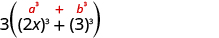Write it using the sum of cubes pattern.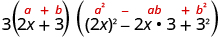Is the expression factored completely? Yes. $3\left(2x+3\right)\left(4{x}^{2}-6x+9\right)$ Check by multiplying. We leave the check to you.

Factor completely: $250{m}^{3}+432$ .

$2\left(5m+6\right)\left(25{m}^{2}-30m+36\right)$

Factor completely: $81{q}^{3}+192$ .

$81\left(q+2\right)\left({q}^{2}-2q+4\right)$

Factor completely: $2{x}^{4}-32$ .

## Solution

$\begin{array}{ccccccc}\text{Is there a GCF?}\hfill & & & \text{Yes, 2.}\hfill & & & \hfill 2{x}^{4}-32\hfill \\ \text{Factor it out.}\hfill & & & & & & \hfill 2\left({x}^{4}-16\right)\hfill \\ \text{In the parentheses, is it a binomial, trinomial,}\hfill & & & & & & \\ \text{or are there more than three terms?}\hfill & & & \text{Binomial.}\hfill & & & \\ \phantom{\rule{1em}{0ex}}\text{Is it a sum or difference?}\hfill & & & \text{Yes.}\hfill & & & \\ \phantom{\rule{1em}{0ex}}\text{Of squares or cubes?}\hfill & & & \text{Difference of squares.}\hfill & & & \hfill 2\left({\left({x}^{2}\right)}^{2}-{\left(4\right)}^{2}\right)\hfill \\ \text{Write it as a product of conjugates.}\hfill & & & & & & \hfill 2\left({x}^{2}-4\right)\left({x}^{2}+4\right)\hfill \\ \text{The first binomial is again a difference of squares.}\hfill & & & & & & \hfill 2\left({\left(x\right)}^{2}-{\left(2\right)}^{2}\right)\left({x}^{2}+4\right)\hfill \\ \text{Write it as a product of conjugates.}\hfill & & & & & & \hfill 2\left(x-2\right)\left(x+2\right)\left({x}^{2}+4\right)\hfill \\ \text{Is the expression factored completely?}\hfill & & & \text{Yes.}\hfill & & & \\ \\ \\ \phantom{\rule{1em}{0ex}}\text{None of these binomials is a difference of squares.}\hfill & & & & & & \\ \text{Check your answer.}\hfill & & & & & & \\ \\ \\ \phantom{\rule{1em}{0ex}}\text{Multiply.}\hfill & & & & & & \\ \\ \\ \\ \\ \\ \phantom{\rule{3em}{0ex}}\begin{array}{c}2\left(x-2\right)\left(x+2\right)\left({x}^{2}+4\right)\hfill \\ 2\left({x}^{2}-4\right)\left({x}^{2}+4\right)\hfill \\ 2\left({x}^{4}-16\right)\hfill \\ 2{x}^{4}-32✓\hfill \end{array}\hfill & & & & & & \end{array}$

Factor completely: $4{a}^{4}-64$ .

$4\left({a}^{2}+4\right)\left(a-2\right)\left(a+2\right)$

Factor completely: $7{y}^{4}-7$ .

$7\left({y}^{2}+1\right)\left(y-1\right)\left(y+1\right)$

Factor completely: $3{x}^{2}+6bx-3ax-6ab$ .

## Solution

$\begin{array}{ccccccc}\text{Is there a GCF?}\hfill & & & \text{Yes, 3.}\hfill & & & \hfill 3{x}^{2}+6bx-3ax-6ab\hfill \\ \\ \\ \text{Factor out the GCF.}\hfill & & & & & & \hfill 3\left({x}^{2}+2bx-ax-2ab\right)\hfill \\ \\ \\ \text{In the parentheses, is it a binomial, trinomial,}\hfill & & & \text{More than 3}\hfill & & & \\ \text{or are there more terms?}\hfill & & & \text{terms.}\hfill & & & \\ \\ \\ \text{Use grouping.}\hfill & & & & & & \hfill 3\left[x\left(x+2b\right)-a\left(x+2b\right)\right]\hfill \\ & & & & & & \hfill 3\left(x+2b\right)\left(x-a\right)\hfill \\ \text{Check your answer.}\hfill & & & & & & \\ \\ \\ \phantom{\rule{1em}{0ex}}\text{Is the expression factored completely? Yes.}\hfill & & & & & & \\ \phantom{\rule{1em}{0ex}}\text{Multiply.}\hfill & & & & & & \\ \phantom{\rule{3em}{0ex}}3\left(x+2b\right)\left(x-a\right)\hfill & & & & & & \\ \phantom{\rule{3em}{0ex}}3\left({x}^{2}-ax+2bx-2ab\right)\hfill & & & & & & \\ \phantom{\rule{3em}{0ex}}3{x}^{2}-3ax+6bx-6ab\phantom{\rule{0.2em}{0ex}}✓\hfill & & & & & & \end{array}$

Factor completely: $6{x}^{2}-12xc+6bx-12bc$ .

$6\left(x+b\right)\left(x-2c\right)$

Factor completely: $16{x}^{2}+24xy-4x-6y$ .

$2\left(4x-1\right)\left(x+3y\right)$

Factor completely: $10{x}^{2}-34x-24$ .

## Solution

$\begin{array}{ccccccc}\text{Is there a GCF?}\hfill & & & \text{Yes, 2.}\hfill & & & \hfill 10{x}^{2}-34x-24\hfill \\ \\ \\ \text{Factor out the GCF.}\hfill & & & & & & \hfill 2\left(5{x}^{2}-17x-12\right)\hfill \\ \\ \\ \text{In the parentheses, is it a binomial, trinomial,}\hfill & & & \hfill \text{Trinomial with}\hfill & & & \\ \text{or are there more than three terms?}\hfill & & & a\ne 1.\hfill & & & \\ \\ \\ \text{Use trial and error or the “ac” method.}\hfill & & & & & & \hfill 2\underset{}{\left(5{x}^{2}}-17x\underset{}{-12\right)}\hfill \\ & & & & & & \hfill 2\left(5x+3\right)\left(x-4\right)\hfill \\ \\ \\ \begin{array}{c}\text{Check your answer. Is the expression factored}\hfill \\ \text{completely? Yes.}\hfill \end{array}\hfill & & & & & & \\ \\ \\ \phantom{\rule{2em}{0ex}}\text{Multiply.}\hfill & & & & & & \\ \phantom{\rule{3em}{0ex}}2\left(5x+3\right)\left(x-4\right)\hfill & & & & & & \\ \phantom{\rule{3em}{0ex}}2\left(5{x}^{2}-20x+3x-12\right)\hfill & & & & & & \\ \phantom{\rule{3em}{0ex}}2\left(5{x}^{2}-17x-12\right)\hfill & & & & & & \\ \phantom{\rule{3em}{0ex}}10{x}^{2}-34x-24\phantom{\rule{0.2em}{0ex}}✓\hfill & & & & & & \end{array}$

Factor completely: $4{p}^{2}-16p+12$ .

$4\left(p-1\right)\left(p-3\right)$

Factor completely: $6{q}^{2}-9q-6$ .

$3\left(q-2\right)\left(2q+1\right)$

## Key concepts

• General Strategy for Factoring Polynomials See [link] .
• How to Factor Polynomials
1. Is there a greatest common factor? Factor it out.
2. Is the polynomial a binomial, trinomial, or are there more than three terms?
• If it is a binomial:
Is it a sum?
• Of squares? Sums of squares do not factor.
• Of cubes? Use the sum of cubes pattern.
Is it a difference?
• Of squares? Factor as the product of conjugates.
• Of cubes? Use the difference of cubes pattern.
• If it is a trinomial:
Is it of the form ${x}^{2}+bx+c$ ? Undo FOIL.
Is it of the form $a{x}^{2}+bx+c$ ?
• If ‘a’ and ‘c’ are squares, check if it fits the trinomial square pattern.
• Use the trial and error or ‘ac’ method.
• If it has more than three terms:
Use the grouping method.
3. Check. Is it factored completely? Do the factors multiply back to the original polynomial?

## Practice makes perfect

Recognize and Use the Appropriate Method to Factor a Polynomial Completely

In the following exercises, factor completely.

$10{x}^{4}+35{x}^{3}$

$5{x}^{3}\left(2x+7\right)$

$18{p}^{6}+24{p}^{3}$

${y}^{2}+10y-39$

$\left(y-3\right)\left(y+13\right)$

${b}^{2}-17b+60$

$2{n}^{2}+13n-7$

$\left(2n-1\right)\left(n+7\right)$

$8{x}^{2}-9x-3$

${a}^{5}+9{a}^{3}$

${a}^{3}\left({a}^{2}+9\right)$

$75{m}^{3}+12m$

$121{r}^{2}-{s}^{2}$

$\left(11r-s\right)\left(11r+s\right)$

$49{b}^{2}-36{a}^{2}$

$8{m}^{2}-32$

$8\left(m-2\right)\left(m+2\right)$

$36{q}^{2}-100$

$25{w}^{2}-60w+36$

${\left(5w-6\right)}^{2}$

$49{b}^{2}-112b+64$

${m}^{2}+14mn+49{n}^{2}$

${\left(m+7n\right)}^{2}$

$64{x}^{2}+16xy+{y}^{2}$

$7{b}^{2}+7b-42$

$7\left(b+3\right)\left(b-2\right)$

$3{n}^{2}+30n+72$

$3{x}^{3}-81$

$3\left(x-3\right)\left({x}^{2}+3x+9\right)$

$5{t}^{3}-40$

${k}^{4}-16$

$\left(k-2\right)\left(k+2\right)\left({k}^{2}+4\right)$

${m}^{4}-81$

$15pq-15p+12q-12$

$3\left(5p+4\right)\left(q-1\right)$

$12ab-6a+10b-5$

$4{x}^{2}+40x+84$

$4\left(x+3\right)\left(x+7\right)$

$5{q}^{2}-15q-90$

${u}^{5}+{u}^{2}$

${u}^{2}\left(u+1\right)\left({u}^{2}-u+1\right)$

$5{n}^{3}+320$

$4{c}^{2}+20cd+81{d}^{2}$

prime

$25{x}^{2}+35xy+49{y}^{2}$

$10{m}^{4}-6250$

$10\left(m-5\right)\left(m+5\right)\left({m}^{2}+25\right)$

$3{v}^{4}-768$

## Everyday math

Watermelon drop A springtime tradition at the University of California San Diego is the Watermelon Drop, where a watermelon is dropped from the seventh story of Urey Hall.

1. The binomial $-16{t}^{2}+80$ gives the height of the watermelon $t$ seconds after it is dropped. Factor the greatest common factor from this binomial.
2. If the watermelon is thrown down with initial velocity 8 feet per second, its height after $t$ seconds is given by the trinomial $-16{t}^{2}-8t+80$ . Completely factor this trinomial.

$-16\left({t}^{2}-5\right)$ $-8\left(2t+5\right)\left(t-2\right)$

Pumpkin drop A fall tradition at the University of California San Diego is the Pumpkin Drop, where a pumpkin is dropped from the eleventh story of Tioga Hall.

1. The binomial $-16{t}^{2}+128$ gives the height of the pumpkin t seconds after it is dropped. Factor the greatest common factor from this binomial.
2. If the pumpkin is thrown down with initial velocity 32 feet per second, its height after $t$ seconds is given by the trinomial $-16{t}^{2}-32t+128$ . Completely factor this trinomial.

## Writing exercises

The difference of squares ${y}^{4}-625$ can be factored as $\left({y}^{2}-25\right)\left({y}^{2}+25\right)$ . But it is not completely factored. What more must be done to completely factor it?

Of all the factoring methods covered in this chapter (GCF, grouping, undo FOIL, ‘ac’ method, special products) which is the easiest for you? Which is the hardest? Explain your answers.

## Self check

After completing the exercises, use this checklist to evaluate your mastery of the objectives of this section.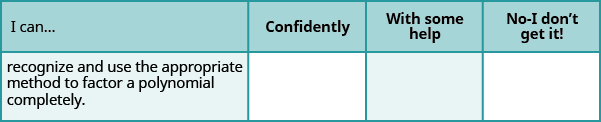Overall, after looking at the checklist, do you think you are well-prepared for the next section? Why or why not?

Macario is making 12 pounds of nut mixture with macadamia nuts and almonds. macadamia nuts cost $9 per pound and almonds cost$5.25 per pound. how many pounds of macadamia nuts and how many pounds of almonds should macario use for the mixture to cost $6.50 per pound to make? Cherry Reply Nga and Lauren bought a chest at a flea market for$50. They re-finished it and then added a 350 % mark - up
the sum of two Numbers is 19 and their difference is 15
2, 17
Jose
interesting
saw
Felecia left her home to visit her daughter, driving 45mph. Her husband waited for the dog sitter to arrive and left home 20 minutes, or 13 hour later. He drove 55mph to catch up to Felecia. How long before he reaches her?
integer greater than 2 and less than 12
2 < x < 12
Felix
I'm guessing you are doing inequalities...
Felix
Actually, translating words into algebraic expressions / equations...
Felix
hi
Darianna
hello
Mister
Eric here
Eric
He charges $125 per job. His monthly expenses are$1,600. How many jobs must he work in order to make a profit of at least $2,400? Alicia Reply at least 20 Ayla what are the steps? Alicia 6.4 jobs Grahame 32 Grahame 1600+2400= total amount with expenses. 4000/125= number of jobs needed to make that min profit of 2400. answer is 32 Orlando He must work 32 jobs to make a profit POP what is algebra Azhar Reply repeated addition and subtraction of the order of operations. i love algebra I'm obsessed. Shemiah hi Krekar Eric here. I'm a parent. 53 years old. I have never taken algebra. I want to learn. Eric One-fourth of the candies in a bag of M&M’s are red. If there are 23 red candies, how many candies are in the bag? Leanna Reply they are 92 candies in the bag POP rectangular field solutions Navin Reply What is this? Donna t muqtaar the proudact of 3x^3-5×^2+3 and 2x^2+5x-4 in z7[x]/ is anas Reply ? Choli a rock is thrown directly upward with an initial velocity of 96feet per second from a cliff 190 feet above a beach. The hight of tha rock above the beach after t second is given by the equation h=_16t^2+96t+190 Usman Stella bought a dinette set on sale for$725. The original price was $1,299. To the nearest tenth of a percent, what was the rate of discount? Manhwa Reply 44.19% Scott 40.22% Terence 44.2% Orlando I don't know Donna if you want the discounted price subtract$725 from $1299. then divide the answer by$1299. you get 0.4419... but as percent you get 44.19... but to the nearest tenth... round .19 to .2 and you get 44.2%
Orlando
you could also just divide $725/$1299 and then subtract it from 1. then you get the same answer.
Orlando
p mulripied-5 and add 30 to it
Tausif
Tausif
how
muqtaar
Can you explain further
p mulripied-5 and add to 30
Tausif
-5p+30?
Corey
p=-5+30
Jacob
How do you find divisible numbers without a calculator?
TAKE OFF THE LAST DIGIT AND MULTIPLY IT 9. SUBTRACT IT THE DIGITS YOU HAVE LEFT. IF THE ANSWER DIVIDES BY 13(OR IS ZERO), THEN YOUR ORIGINAL NUMBER WILL ALSO DIVIDE BY 13!IS DIVISIBLE BY 13
BAINAMA
When she graduates college, Linda will owe $43,000 in student loans. The interest rate on the federal loans is 4.5% and the rate on the private bank loans is 2%. The total interest she owes for one year was$1,585. What is the amount of each loan?By Royalle MooreBy OpenStaxBy Marion CabalfinBy OpenStaxBy OpenStaxBy Gerr ZenBy OpenStaxBy Rachel WoolardBy OpenStaxBy Kevin Moquin